## Euler Parameters

The four parameters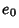,,, anddescribing a finite rotation about an arbitrary axis. The Euler parameters are defined by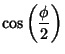(1)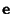(2)

and are a Quaternion in scalar-vector representation(3)

Because Euler's Rotation Theorem states that an arbitrary rotation may be described by only three parameters, a relationship must exist between these four quantities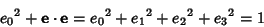(4)

(Goldstein 1980, p. 153). The rotation angle is then related to the Euler parameters by(5)(6)

The Euler parameters may be given in terms of the Euler Angles by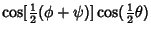(7)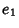(8)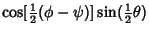(9)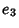(10)

(Goldstein 1980, p. 155).

Using the Euler parameters, the Rotation Formula becomes(11)

and the Rotation Matrix becomes(12)

where the elements of the matrix are(13)

Here, Einstein Summation has been used,is the Kronecker Delta, and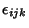is the Permutation Symbol. Written out explicitly, the matrix elements are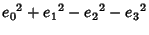(14)(15)(16)(17)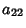(18)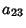(19)(20)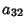(21)(22)

References

Arfken, G. Mathematical Methods for Physicists, 3rd ed. Orlando, FL: Academic Press, pp. 198-200, 1985.